# Know the GMAT Code: Quadratics

by , Oct 8, 2016Youve studied the math, but how well do you know how to decipher unusual math clues that the test writers hide in GMAT problems? To get a really high score on this test, youve got to Know the Code in order to make your way through the toughest problems.

If this is your first introduction to the Know the Code idea, follow the link in that last paragraph to learn more. Then, come back here and test your skills on this problem from the free GMATPrep exams.

Here you go:

*If two of the four expressions [pmath]x + y[/pmath], [pmath]x + 5y[/pmath], [pmath]x-y[/pmath], and [pmath]5x-y[/pmath] are chosen at random, what is the probability that their product will be of the form of [pmath]x^2-(by)^2[/pmath], where [pmath]b[/pmath] is an integer?

(A) [pmath]1/2[/pmath]

(B) [pmath]1/3[/pmath]

(C) [pmath]1/4[/pmath]

(D) [pmath]1/5[/pmath]

(E) [pmath]1/6[/pmath]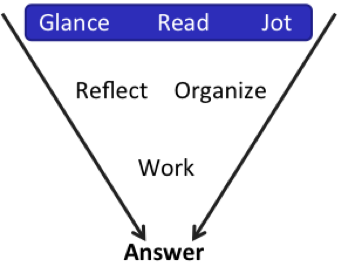Glance at the problem. PS. Lots of text. Variables and some squares algebra? Time to Read and Jot.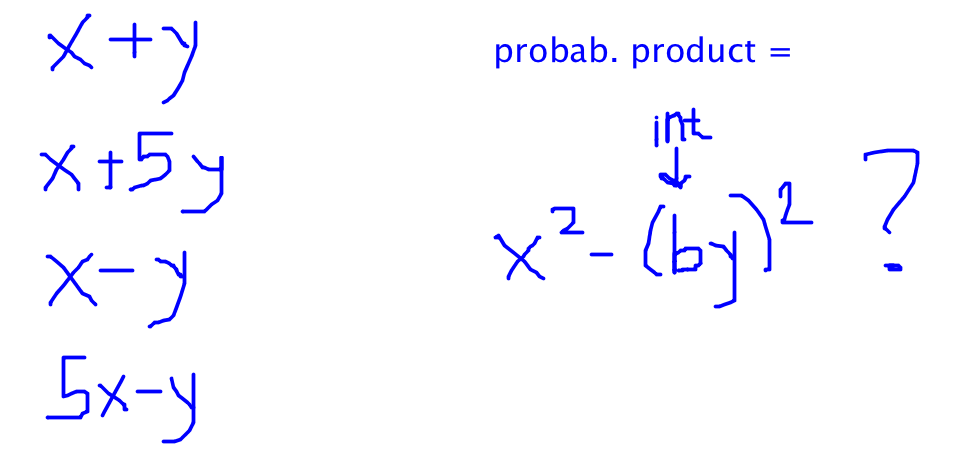All right, lets think about what weve got here.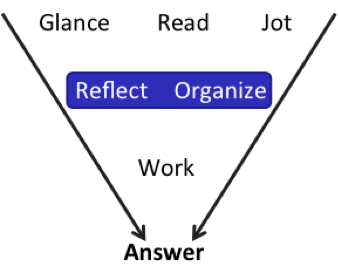Product means Ive got to multiply something together. Oh, I see: I multiple any two of the terms on the left, and thats going to give me something in a quadratic form (except not with an = 0 on the other side). And Im looking to match the form specified in the question.

Heres where you need to know something about quadratics that you may never have realized before.

For two of the common quadratic identities, you get 3 terms, like this:

[pmath](a+b)(a+b)=a^2+2ab+b^2[/pmath]

[pmath](a-b)(a-b)=a^2-2ab+b^2[/pmath]

But there is that one form where you get only two terms:

[pmath](a+b)(a-b)=a^2-b^2[/pmath]

How come that third one has only two terms? Where did the middle term go?

This only works when the Outer and Inner steps of FOIL cancel each other out. That third quadratic really plays out like this:

[pmath](a+b)(a-b)=a^2+ab-ab-b^2=a^2-b^2[/pmath]

What is it that allows those two middle terms to cancel out?

First, one has to be positive and one has to be negative. The positive and negative signs come from the two starting terms: one of those has to be addition and the other one has to be subtraction.

After that, one more thing has to happen. The coefficients have to match up so that the two middle terms exactly cancel out to 0.

Check out what happens in these examples:

[pmath](a + 3b)(a-b)[/pmath]

[pmath](a + b)(3a-b)[/pmath]

[pmath](a + 3b)(3a-b)[/pmath]

[pmath](3a + 3b)(a-b)[/pmath]

Ready? Heres what happens in each case:

[pmath](a+3b)(a-b)=a^2+3ab-ab-3b^2=a^2+2ab-3b^2[/pmath]

[pmath](a+b)(3a-b)=3a^2+3ab-ab-b^2=3a^2+2ab-b^2[/pmath]

[pmath](a+3b)(3a-b)=3a^2+9ab-ab-3b^2=3a^2+8ab-3b^2[/pmath]

[pmath](3a+3b)(a-b)=3a^2+3ab-3ab-b^2=3a^2-3b^2[/pmath]

Only in the fourth case did the two middle terms exactly cancel out. How come?

It turns out that you have to have the same coefficients for[pmath]a[/pmath]and [pmath]b[/pmath]in the same set of parentheses. In the fourth example, each variable has a coefficient of 3 in the first set of parentheses and each variable has a coefficient of 1 in the second set of parentheses. If theyre not the same within each set of parentheses, then the Outer and Inner steps of FOIL wont exactly cancel out.

Have you ever noticed that before? Well, you know now! :D Add that to your Know the Code flash cards.

Okay, knowing what you know now, lets actually do this problem.To calculate probability, you have to find the number of desired outcomes divided by the total number of possible outcomes. Start with the second piece. How many ways are there to multiply together two of these four terms?

You can calculate this using combinatorics principles or you can just count it out. (I hate combinatorics. Im going to count it out!)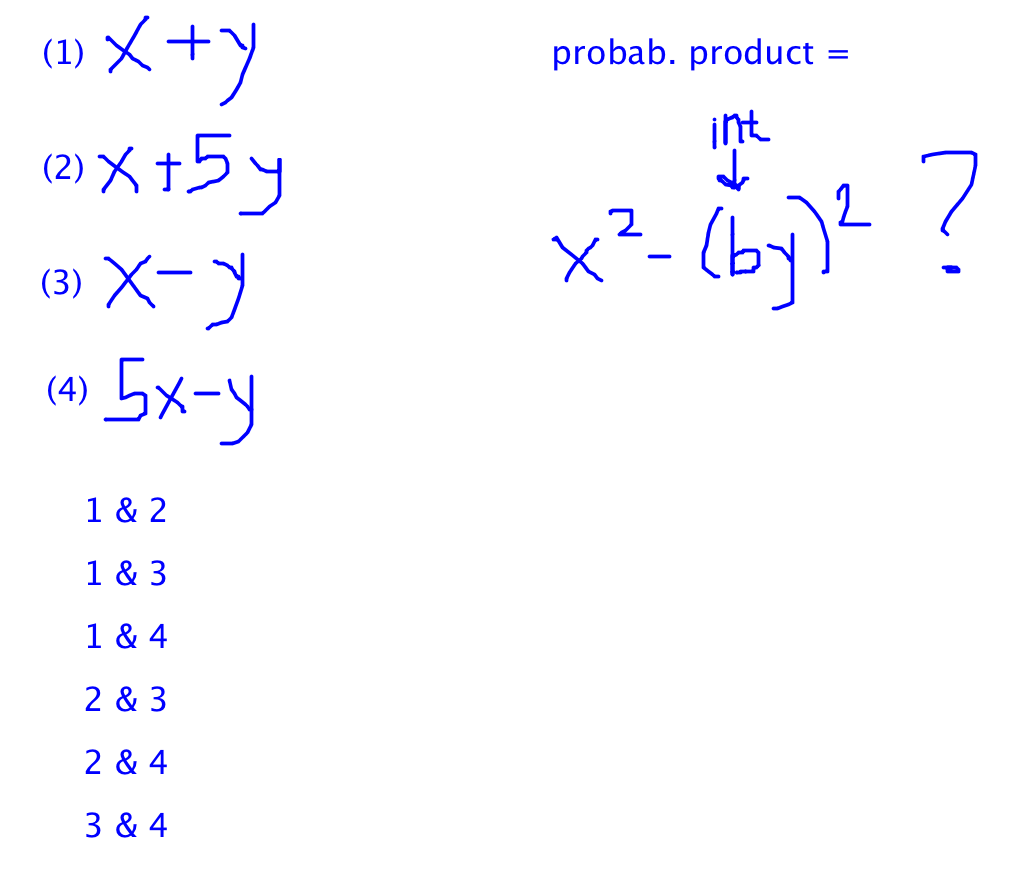To save some effort, I labeled each statement with a number and then wrote out the combinations that way. There are 6 possible combinations.

Now, which of those combinations are going to get the two middle terms to cancel out completely?

First, I need to make sure that Ive got one addition term and one subtraction term.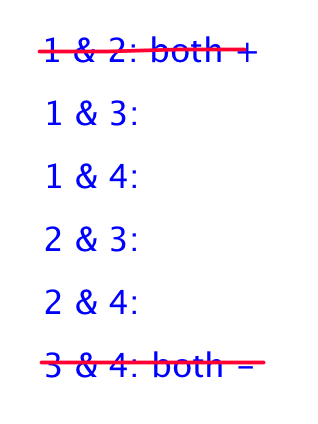The first and last cases dont qualify. Cross them off.

Next, note that the problem asks for the answer to be in the form [pmath]x^2-(by)^2[/pmath]. The [pmath]x^2[/pmath] term doesnt have a number or variable in front of it; in other words, the [pmath]x^2[/pmath]term has to have a coefficient of 1. Cross off any cases that wont have a coefficient of 1 for[pmath]x^2[/pmath]. The term I labeled (4), [pmath]5x-y[/pmath], wont work, since none of the other terms starts out [pmath](1/5)x[/pmath], so cross off any cases that use term 4.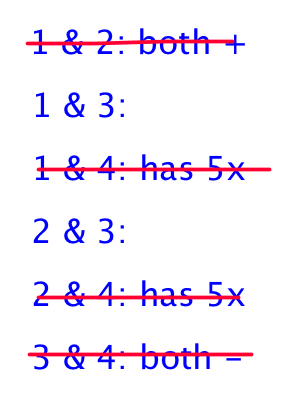Now, make sure that each set of parentheses has the same coefficients for the variables inside of those parentheses. The second term, [pmath]x + 5y[/pmath], doesnt qualify.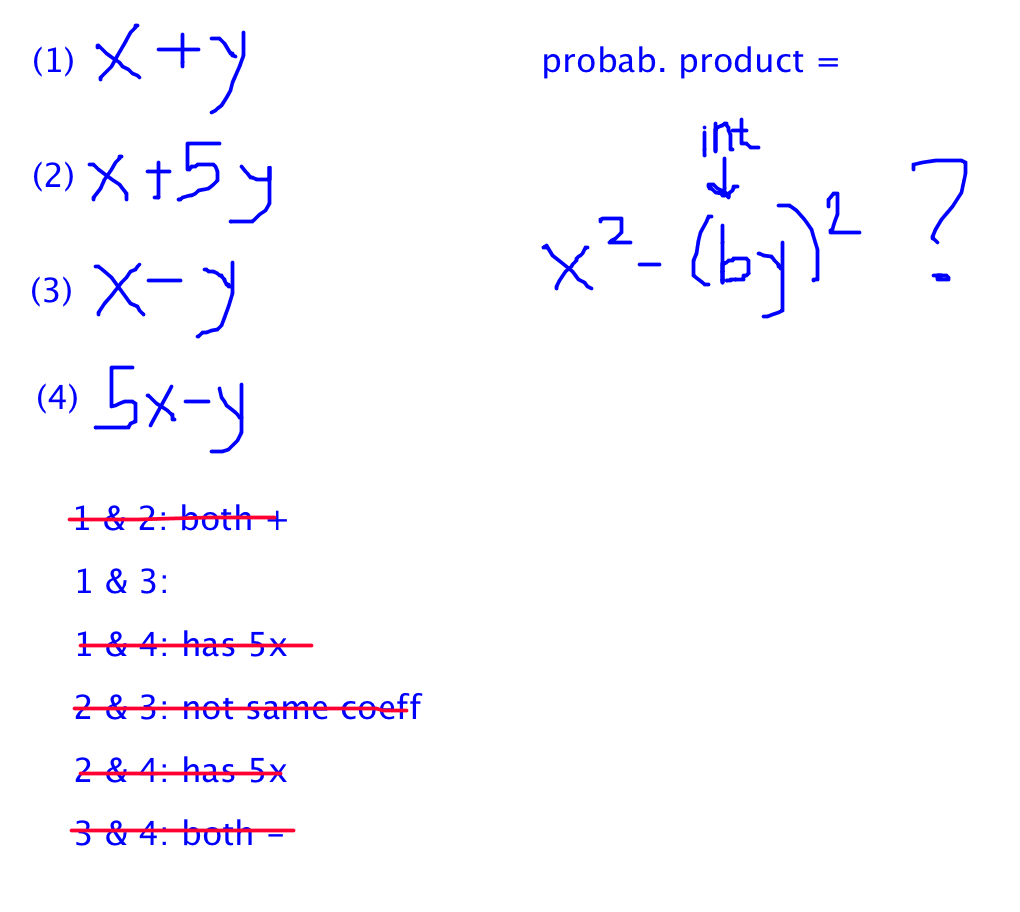The only case that works is 1 & 3:

[pmath](x+y)(x-y)=x^2-y^2[/pmath]

There is 1 desired case out of a total of 6 possible cases, so the probability is 1/6.

Now you could just start trying to multiply out all of the different cases and stop whenever you realize a particular case is going to fail the test. Thats going to take a while though; you can shortcut that process by using the rules that will have to apply in order to reach the desired outcome.

Even if you dont know all of the rules, you can save some time (or at least cross off some answers) by knowing some of them. The most common one is the fact that the two signs will have to be opposite. The coefficient stuff is a bit more complicated, but if youre going for an exceptionally high score on this test, now you can Know the Code.

In order for the common quadratic identity[pmath](a+b)(a-b)=a^2-b^2[/pmath] to have no middle term, the coefficients within each set of parentheses have to be the same. For example: [pmath](3a + 3b)(a-b)[/pmath] works, as does [pmath](2a + 2b)(5a-5b)[/pmath].

## Key Takeaways for Knowing the Code:

(1) When youre studying, dont just stop when you see the textbook answer to a problem. Push yourself to find the patterns or to articulate the underlying principles behind what youre seeing.

(2) Then turn that knowledge into Know the Code flash cards: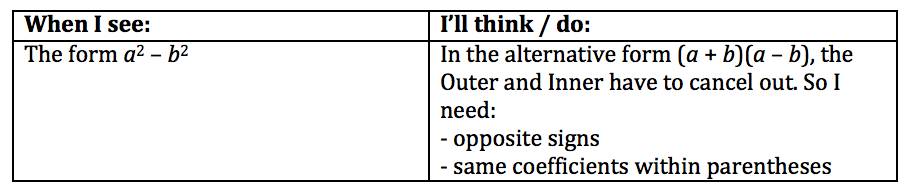* GMATPrep questions courtesy of the Graduate Management Admissions Council. Usage of this question does not imply endorsement by GMAC.